# NCERT Exemplar Solutions for Class 9 Maths Chapter 5 - Introduction To Euclids Geometry

## NCERT Exemplar Solutions Class 9 Maths Chapter 5 – Free PDF Download

NCERT Exemplar Class 9 Mathematics Chapter 5 Introduction to Euclid’s Geometry, is provided herein PDF format for students to prepare for exams. Our experts have designed Exemplar problems with solutions in accordance with the CBSE syllabus for the 9th standard. The NCERT Exemplar questions are provided in such a way that after solving the exemplar questions and exercise questions students will be able to solve difficult questions related to Euclid’s Geometry. To get NCERT exemplar questions and solutions of Class 9 Maths Chapter 5, click on the link below. NCERT Exemplar for Class 9 Maths Chapter 5 covers the following important topics in Euclids Geometry, such as

• Euclid’s Definitions for solids, lines, point, etc.
• Some of Euclid’s axioms
• Problems based on Euclid’s five postulates
• Two equivalent versions of Euclid’s fifth postulate

To provide easy learning and help students to understand the concepts of geometry according to the great Mathematician Euclid’s, free NCERT Exemplars are provided here. These exemplar solutions can be used as a reference tool by 9th standard students while practising the NCERT book exercise questions, which can also be downloaded in PDF form.

## Download PDF of NCERT Exemplar Solutions for Class 9 Maths Chapter 5 Introduction to Euclid’s Geometry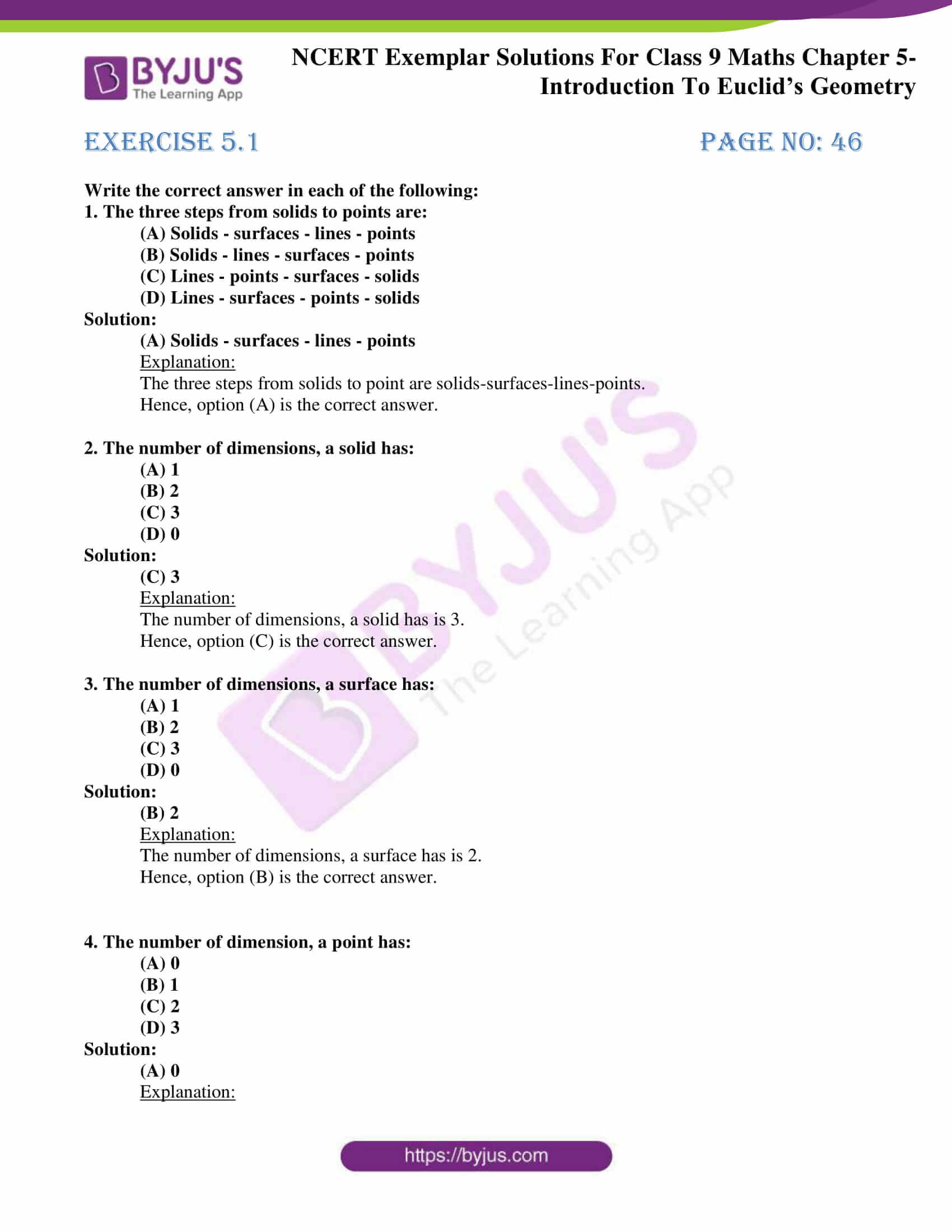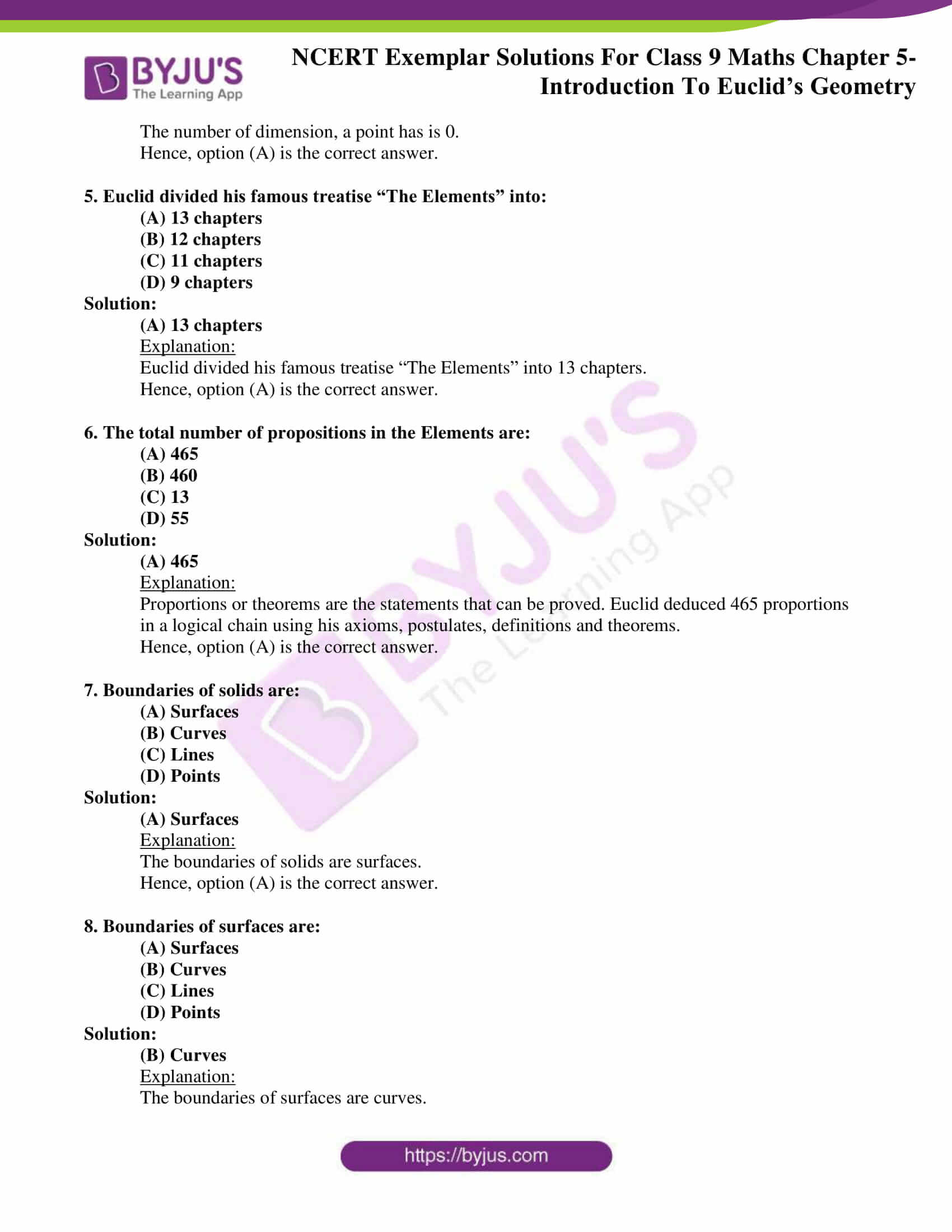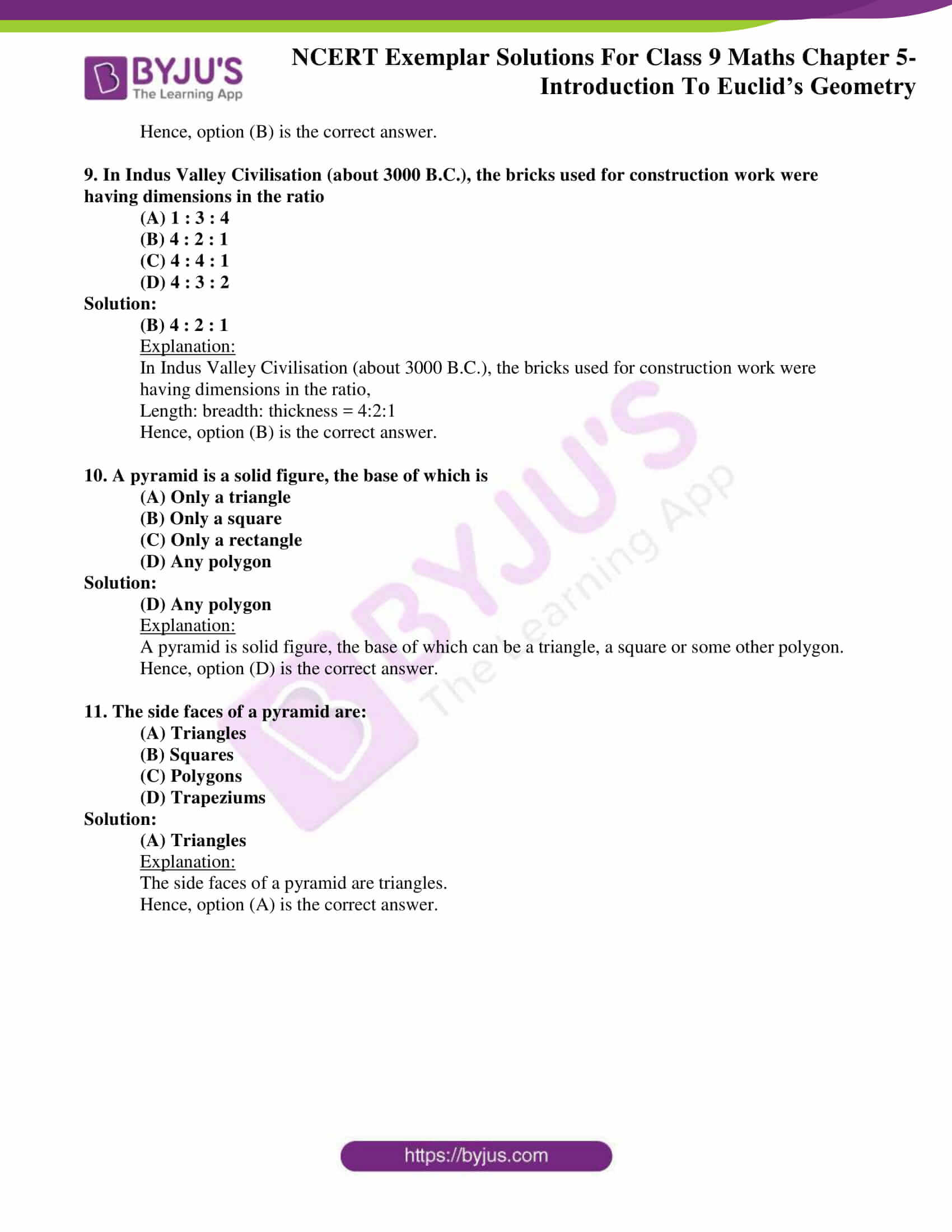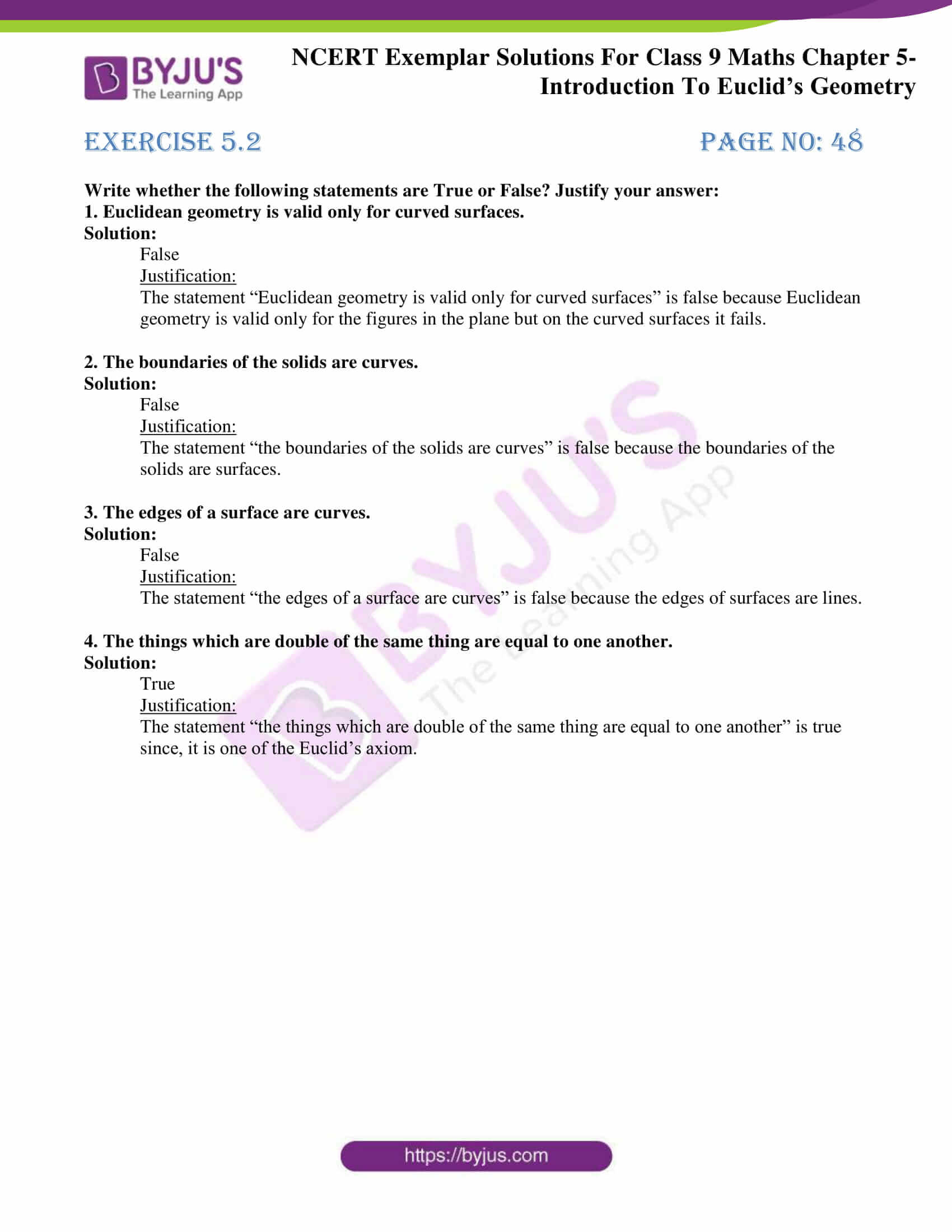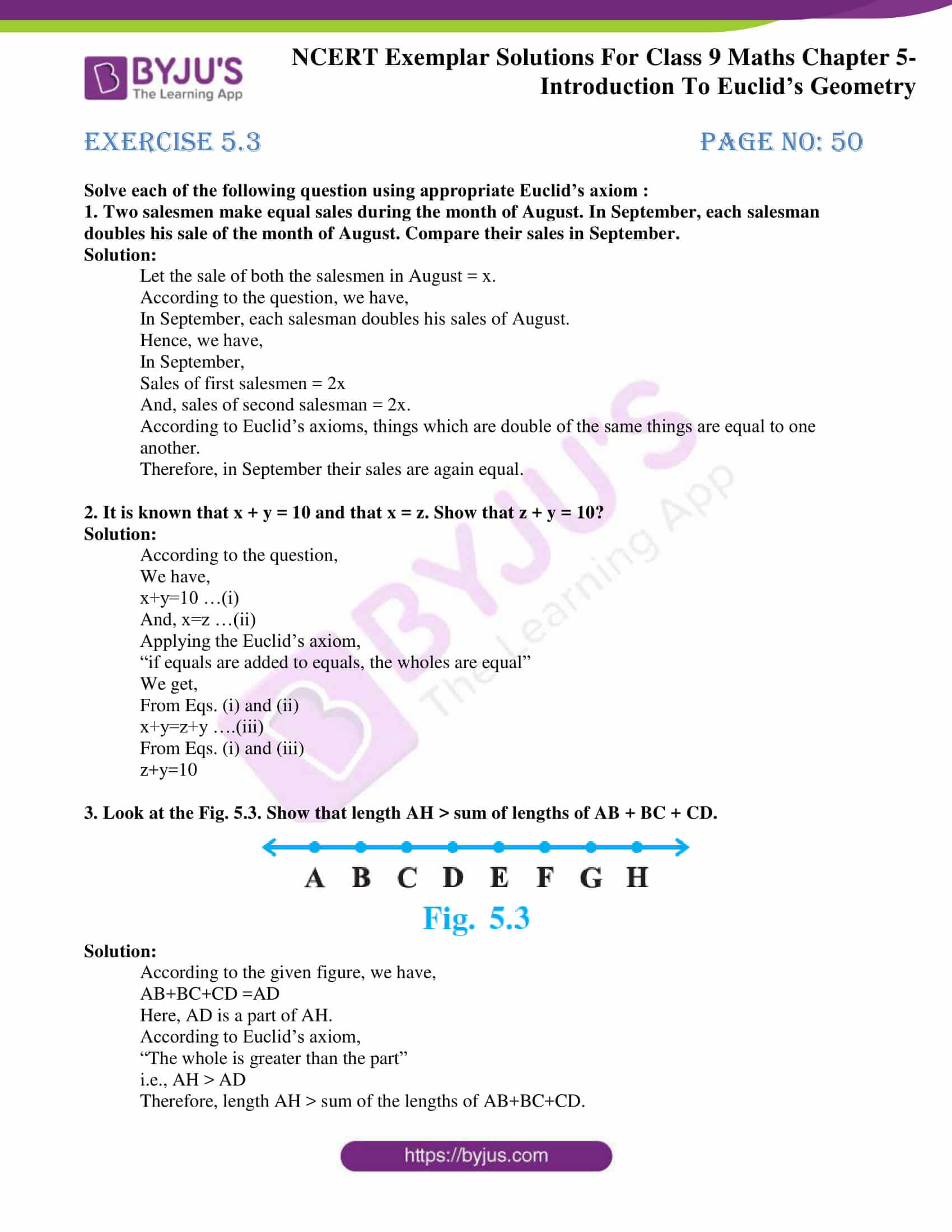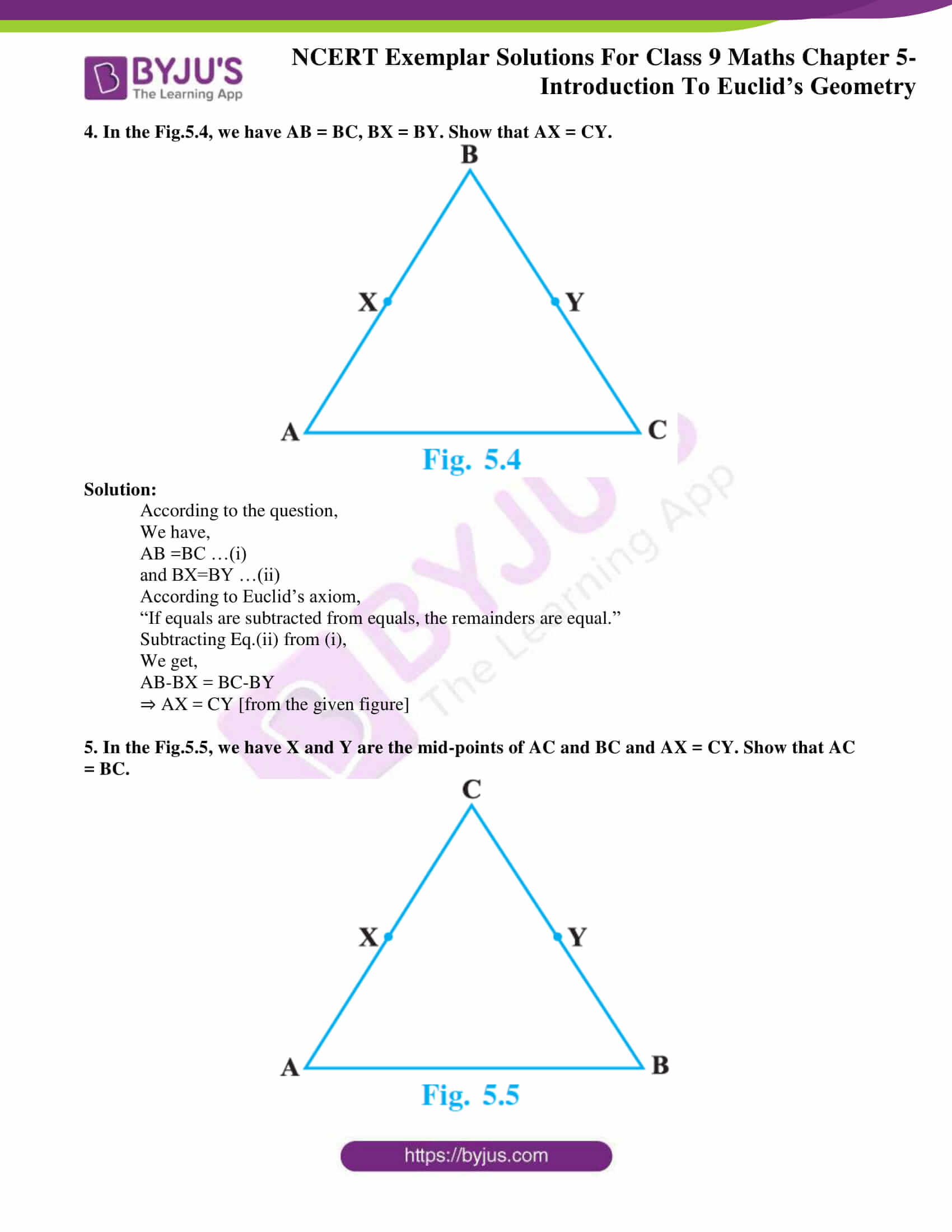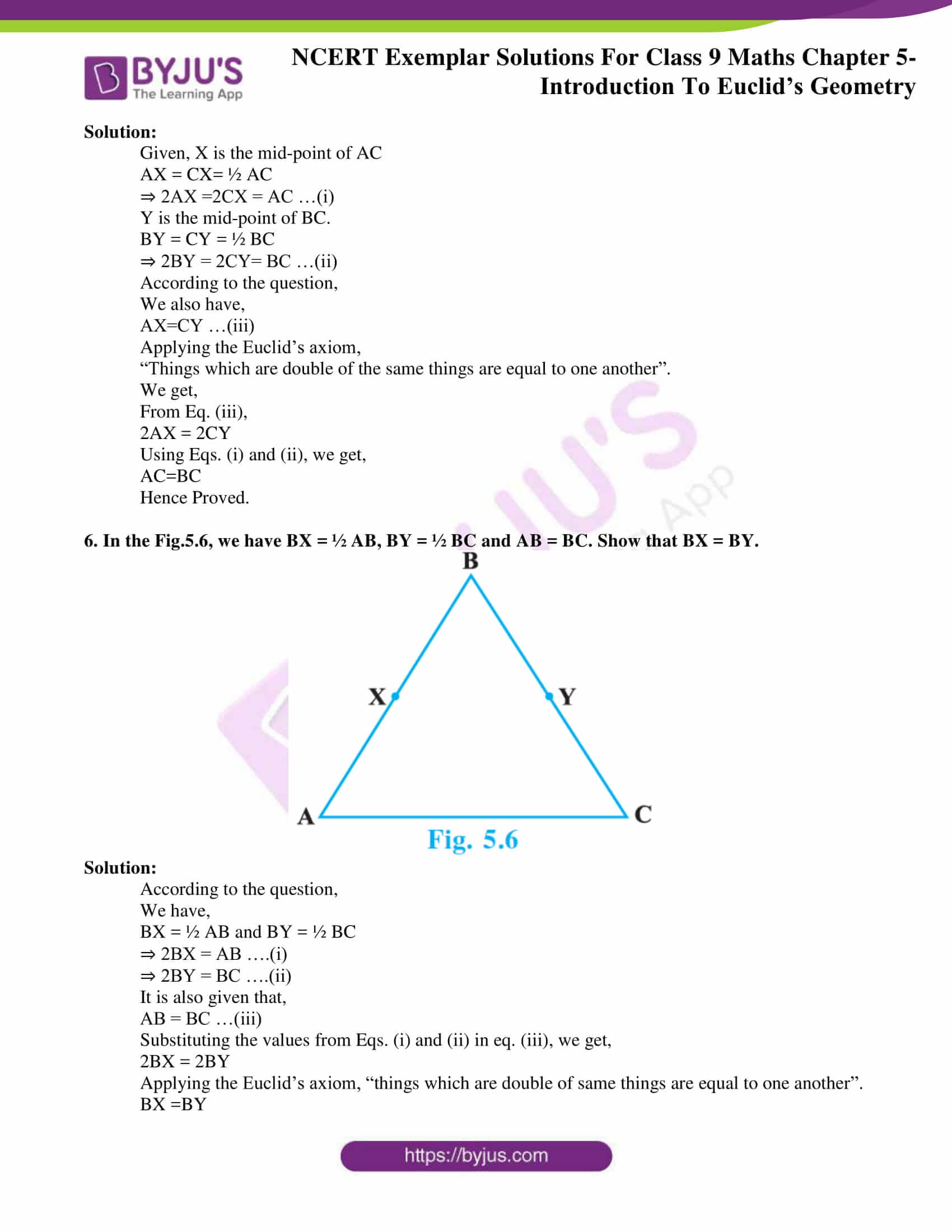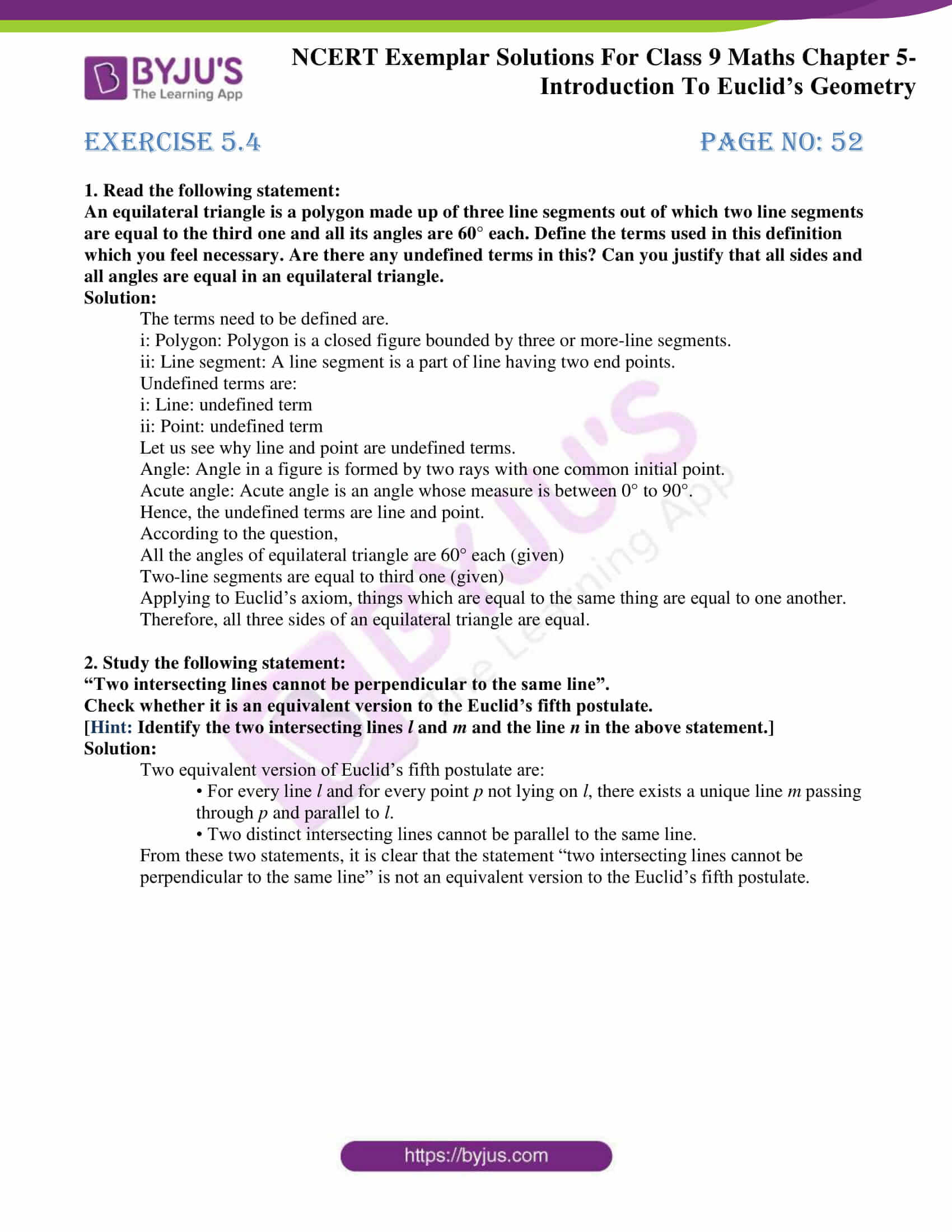### Access Answers to NCERT Exemplar Solutions for Class 9 Maths Chapter 5 Introduction to Euclid’s Geometry

Exercise 5.1 Page No: 46

Write the correct answer in each of the following:

1. The three steps from solids to points are:

(A) Solids – surfaces – lines – points

(B) Solids – lines – surfaces – points

(C) Lines – points – surfaces – solids

(D) Lines – surfaces – points – solids

Solution:

(A) Solids – surfaces – lines – points

Explanation:

The three steps from solids to point are solids-surfaces-lines-points.

Hence, option (A) is the correct answer.

2. The number of dimensions, a solid has:

(A) 1

(B) 2

(C) 3

(D) 0

Solution:

(C) 3

Explanation:

The number of dimensions, a solid has is 3.

Hence, option (C) is the correct answer.

3. The number of dimensions, a surface has:

(A) 1

(B) 2

(C) 3

(D) 0

Solution:

(B) 2

Explanation:

The number of dimensions, a surface has is 2.

Hence, option (B) is the correct answer.

4. The number of dimension, a point has:

(A) 0

(B) 1

(C) 2

(D) 3

Solution:

(A) 0

Explanation:

The number of dimension, a point has is 0.

Hence, option (A) is the correct answer.

5. Euclid divided his famous treatise “The Elements” into:

(A) 13 chapters

(B) 12 chapters

(C) 11 chapters

(D) 9 chapters

Solution:

(A) 13 chapters

Explanation:

Euclid divided his famous treatise “The Elements” into 13 chapters.

Hence, option (A) is the correct answer.

6. The total number of propositions in the Elements are:

(A) 465

(B) 460

(C) 13

(D) 55

Solution:

(A) 465

Explanation:

Proportions or theorems are the statements that can be proved. Euclid deduced 465 proportions in a logical chain using his axioms, postulates, definitions and theorems.

Hence, option (A) is the correct answer.

7. Boundaries of solids are:

(A) Surfaces

(B) Curves

(C) Lines

(D) Points

Solution:

(A) Surfaces

Explanation:

The boundaries of solids are surfaces.

Hence, option (A) is the correct answer.

8. Boundaries of surfaces are:

(A) Surfaces

(B) Curves

(C) Lines

(D) Points

Solution:

(B) Curves

Explanation:

The boundaries of surfaces are curves.

Hence, option (B) is the correct answer.

9. In Indus Valley Civilisation (about 3000 B.C.), the bricks used for construction work were having dimensions in the ratio

(A) 1 : 3 : 4

(B) 4 : 2 : 1

(C) 4 : 4 : 1

(D) 4 : 3 : 2

Solution:

(B) 4 : 2 : 1

Explanation:

In Indus Valley Civilisation (about 3000 B.C.), the bricks used for construction work were having dimensions in the ratio,

Hence, option (B) is the correct answer.

10. A pyramid is a solid figure, the base of which is

(A) Only a triangle

(B) Only a square

(C) Only a rectangle

(D) Any polygon

Solution:

(D) Any polygon

Explanation:

A pyramid is solid figure, the base of which can be a triangle, a square or some other polygon.

Hence, option (D) is the correct answer.

11. The side faces of a pyramid are:

(A) Triangles

(B) Squares

(C) Polygons

(D) Trapeziums

Solution:

(A) Triangles

Explanation:

The side faces of a pyramid are triangles.

Hence, option (A) is the correct answer.

Exercise 5.2 Page No: 48

Write whether the following statements are True or False? Justify your answer:

1. Euclidean geometry is valid only for curved surfaces.

Solution:

False

Justification:

The statement “Euclidean geometry is valid only for curved surfaces” is false because Euclidean geometry is valid only for the figures in the plane but on the curved surfaces it fails.

2. The boundaries of the solids are curves.

Solution:

False

Justification:

The statement “the boundaries of the solids are curves” is false because the boundaries of the solids are surfaces.

3. The edges of a surface are curves.

Solution:

False

Justification:

The statement “the edges of a surface are curves” is false because the edges of surfaces are lines.

4. The things which are double of the same thing are equal to one another.

Solution:

True

Justification:

The statement “the things which are double of the same thing are equal to one another” is true since, it is one of the Euclid’s axiom.

Exercise 5.3 Page No: 50

Solve each of the following question using appropriate Euclid’s axiom :

1. Two salesmen make equal sales during the month of August. In September, each salesman doubles his sale of the month of August. Compare their sales in September.

Solution:

Let the sale of both the salesmen in August = x.

According to the question, we have,

In September, each salesman doubles his sales of August.

Hence, we have,

In September,

Sales of first salesmen = 2x

And, sales of second salesman = 2x.

According to Euclid’s axioms, things which are double of the same things are equal to one another.

Therefore, in September their sales are again equal.

2. It is known that x + y = 10 and that x = z. Show that z + y = 10?

Solution:

According to the question,

We have,

x+y=10 …(i)

And, x=z …(ii)

Applying the Euclid’s axiom,

“if equals are added to equals, the wholes are equal”

We get,

From Eqs. (i) and (ii)

x+y=z+y ….(iii)

From Eqs. (i) and (iii)

z+y=10

3. Look at the Fig. 5.3. Show that length AH > sum of lengths of AB + BC + CD.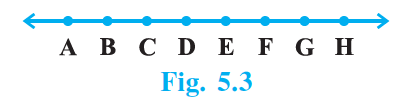Solution:

According to the given figure, we have,

Here, AD is a part of AH.

According to Euclid’s axiom,

“The whole is greater than the part”

Therefore, length AH > sum of the lengths of AB+BC+CD.

4. In the Fig.5.4, we have AB = BC, BX = BY. Show that AX = CY.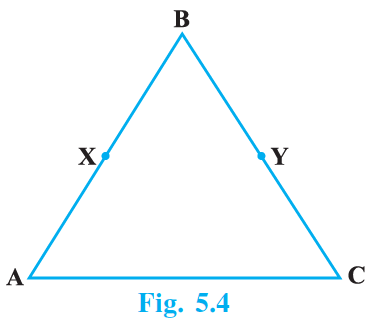Solution:

According to the question,

We have,

AB =BC …(i)

and BX=BY …(ii)

According to Euclid’s axiom,

“If equals are subtracted from equals, the remainders are equal.”

Subtracting Eq.(ii) from (i),

We get,

AB-BX = BC-BY

⇒ AX = CY [from the given figure]

5. In the Fig.5.5, we have X and Y are the mid-points of AC and BC and AX = CY. Show that AC = BC.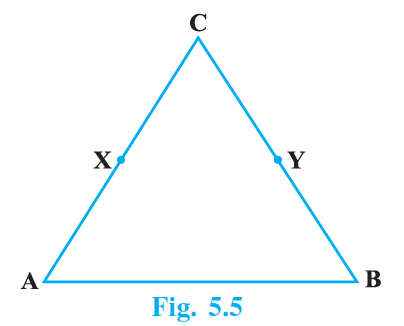Solution:

Given, X is the mid-point of AC

AX = CX= ½ AC

⇒ 2AX =2CX = AC …(i)

Y is the mid-point of BC.

BY = CY = ½ BC

⇒ 2BY = 2CY= BC …(ii)

According to the question,

We also have,

AX=CY …(iii)

Applying the Euclid’s axiom,

“Things which are double of the same things are equal to one another”.

We get,

From Eq. (iii),

2AX = 2CY

Using Eqs. (i) and (ii), we get,

AC=BC

Hence Proved.

6. In the Fig.5.6, we have BX = ½ AB, BY = ½ BC and AB = BC. Show that BX = BY.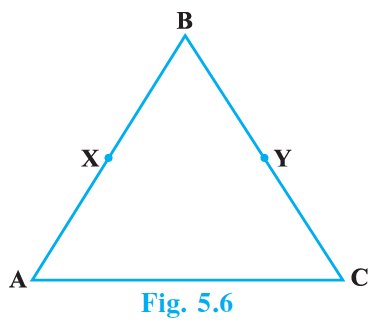Solution:

According to the question,

We have,

BX = ½ AB and BY = ½ BC

⇒ 2BX = AB ….(i)

⇒ 2BY = BC ….(ii)

It is also given that,

AB = BC …(iii)

Substituting the values from Eqs. (i) and (ii) in eq. (iii), we get,

2BX = 2BY

Applying the Euclid’s axiom, “things which are double of same things are equal to one another”.

BX =BY

Exercise 5.4 Page No: 52

An equilateral triangle is a polygon made up of three line segments out of which two line segments are equal to the third one and all its angles are 60° each. Define the terms used in this definition which you feel necessary. Are there any undefined terms in this? Can you justify that all sides and all angles are equal in an equilateral triangle.

Solution:

The terms need to be defined are.

i: Polygon: Polygon is a closed figure bounded by three or more-line segments.

ii: Line segment: A line segment is a part of line having two end points.

Undefined terms are:

i: Line: undefined term

ii: Point: undefined term

Let us see why line and point are undefined terms.

Angle: Angle in a figure is formed by two rays with one common initial point.

Acute angle: Acute angle is an angle whose measure is between 0° to 90°.

Hence, the undefined terms are line and point.

According to the question,

All the angles of equilateral triangle are 60° each (given)

Two-line segments are equal to third one (given)

Applying to Euclid’s axiom, things which are equal to the same thing are equal to one another.

Therefore, all three sides of an equilateral triangle are equal.

2. Study the following statement:

“Two intersecting lines cannot be perpendicular to the same line”.

Check whether it is an equivalent version to the Euclid’s fifth postulate.

[Hint: Identify the two intersecting lines l and m and the line n in the above statement.]

Solution:

Two equivalent version of Euclid’s fifth postulate are:

• For every line l and for every point p not lying on l, there exists a unique line m passing through p and parallel to l.

• Two distinct intersecting lines cannot be parallel to the same line.

From these two statements, it is clear that the statement “two intersecting lines cannot be perpendicular to the same line” is not an equivalent version to the Euclid’s fifth postulate.

Here in BYJU’S, you will also get some more study materials such as exemplar books, NCERT Solutions, notes, and questions papers which can be used to prepare for the exam thoroughly. Solve sample papers and previous year question papers to know questions pattern asked from Maths chapter 5, Euclid’s Geometry and marks contained by it.

Download BYJU’S-The learning app to get personalized videos teaching various concepts of geometry explained by Euclid’s as well as various other concepts with the help of pictures and video contents.

## Frequently Asked Questions on NCERT Exemplar Solutions for Class 9 Maths Chapter 5

### What are the topics covered under NCERT Exemplar Solutions for Class 9 Maths Chapter 5?

The topics covered under NCERT Exemplar Solutions for Class 9 Maths Chapter 5 are
1. Euclid’s Definitions for solids, lines, point, etc.
2. Some of Euclid’s axioms
3. Problems based on Euclid’s five postulates
4. Two equivalent versions of Euclid’s fifth postulate

### What is Euclidean Geometry in NCERT Exemplar Solutions for Class 9 Maths Chapter 5.

Euclidean Geometry is considered an axiomatic system, where all the theorems are derived from a small number of simple axioms. Since the term “Geometry” deals with things like points, lines, angles, squares, triangles, and other shapes, Euclidean Geometry is also known as “plane geometry”.

### Does BYJU’S give the most reliable answers in Chapter 5 of NCERT Exemplar Solutions for Class 9 Maths?

The most accurate and reliable NCERT Exemplar Solutions for Class 9 Maths Chapter 5 are available on BYJU’S. Students can easily download the solutions which are presented in PDF format and access them during their exam preparation. The solutions are framed and compiled by a set of expert faculty who possess numerous years of experience in the respective subjects. The most detailed solutions of the exercise wise problems are curated with the aim of helping students to ace the exam without fear.